### PAPER:

An explicitly solvable multi-scale stochastic volatility model: option pricing and calibration problems

Warning: If your browser does not allow you to see the content of this website click here to download a word file that reproduces the material contained here.

1. Abstract

In  we study  an explicitly solvable multiscale stochastic volatility model that generalizes the Heston model. The model describes the dynamics of an asset price and of its two stochastic variances using a system of three Ito stochastic differential equations. The two stochastic variances vary on two different time scales and can be regarded as auxiliary variables introduced to model the dynamics of the asset price. Under some assumptions the transition probability density function of the stochastic process solution of the model is represented as a one dimensional integral of an explicitly known integrand. In this sense the model is explicitly solvable. In mathematical finance multiscale stochastic volatility models are used for several purposes such as, for example: i) the study of commodity prices, in fact usually commodity prices are characterized by spikes that can be modeled using a fast time scale volatility together with a standard time scale volatility, ii) the study of financial products that live for long time periods (such as life insurance contracts) that can be modeled using a long time scale volatility together with a standard time scale volatility. In alternative to multiscale stochastic volatility models the stochastic jump models, such as, for example, Lévy's processes with stochastic volatility, can be used. However this last type of models is explicitly solvable only in very special circumstances and in general the transition probability density functions associated to them are defined as solutions of integro-differential equations that must be solved numerically. So that the use of jump models in practical situations is, in general, cumbersome when compared to the use of the model proposed here. In order to use the multiscale stochastic volatility model considered to compute option prices we define its risk neutral measure and the associated risk premium parameters. In  we derive a formula for the price of the European vanilla (call and put) options in the multiscale stochastic volatility model. The formulae obtained are given  as one dimensional integrals of explicitly known integrands and in a special case reduce to the corresponding formulae in the Heston model  . This last formula in the case of a European vanilla call option improves the formula given in Heston  formula (10) in a sense made clear later. We use the option price formulae obtained to study the values of the model parameters, of the correlation coefficients of the Wiener processes defining the model and of the initial stochastic variances implied by the ``observed" option prices using both synthetic and real data. The real data analyzed are those relative to the S&P 500 index in the year 2005. That is we generalize to the model proposed here the so called term structure analysis of the implied volatility used in the Black Scholes model. This analysis is translated in the solution of a constrained nonlinear optimization problem with an objective function containing an integral that must be evaluated numerically. The real data analysis presented shows that the multiscale stochastic volatility model gives a satisfactory explanation of the observed option prices including those of the out of the money options and that the implied values of the model parameters, of the correlation coefficients and of the initial stochastic variances can be used to obtain high quality forecasts of the option prices. This  website contains some auxiliary material including some animations that helps the understanding of . A more general reference to the work of the authors and of their coauthors in mathematical finance is the website: http://www.econ.univpm.it/recchioni/finance.

2. Introduction

We present a multiscale stochastic volatility model in mathematical finance. Multiscale modeling is used in a variety of scientific and technical fields and there are many problems that can be described satisfactorily within a multiscale framework (such as, for example, problems in turbulent flow, porous media, weather forecasting, material science, wave propagation, microstructure simulation and biological cell behaviour). However only recently multiscale models have attracted the attention of the mathematical finance community. In  we consider the use of multiscale models in the study of the stochastic volatility associated to an asset price. The idea of using stochastic volatility models to describe the dynamics of an asset price derives from the empirical evidence that the asset price dynamics is driven by processes with non constant volatility. In fact when the constant volatility Black and Scholes model  is used to interpret financial data two phenomena are observed: (i) the volatility “smile” that appears in the volatilities implied by the observed option prices, (ii) the presence of skewness and kurtosis in the asset price probability density function deduced from the empirical data. These phenomena show that the Black Scholes model fails to describe correctly the market behaviour. Several alternatives have been proposed to improve the market description, among them we consider specifically the stochastic volatility models. In these models the stochastic volatility or the stochastic variance of the asset price is defined as the solution of a stochastic differential equation that is coupled with the asset price stochastic differential equation. Models of this type are, for example, the Heston model , that has received special attention since in several circumstances it is able to describe satisfactorily the price dynamics of relevant assets. Furthermore the Heston stochastic volatility model is an explicitly solvable model in the sense that in the Heston model the joint probability density function associated to the asset price and to its stochastic variance can be written as a one dimensional integral of an explicitly known integrand. Heston in  derived a formula for the price of a European vanilla call option in the Heston model given by a one dimensional integral. Later Lipton  pagg. 602-605 derived for the Heston model a representation formula for the joint probability density function of the asset price and of the associated stochastic variance given by a one dimensional oscillatory integral of an explicitly known integrand and he used this formula to price forward starting options. Using the ideas presented in    pagg. 602-605 we have derived in ,  in the Heston model framework, an integral representation formula for the prices of European vanilla options that involves only a one dimensional integral of an explicitly known integrand. In a sense explained in  and summarized here at the end of this Section the formulae derived in  and refined in  ,   are different from that derived in   and are an improvement of the formula derived in .

In the construction of the multiscale stochastic volatility model proposed we start from the fact that several empirical studies of real data have shown that the term structure of the implied volatility of the price of several underlyings (such as, for example, market indices, commodities,....) seems to be driven by two different factors: one fluctuating on a fast time scale and the other fluctuating on a long time scale.  Moreover it has been observed that the financial derivatives with long residual life are not priced satisfactorily by one factor stochastic volatility models such as the Heston model. That is there is empirical evidence of the fact that in the circumstances mentioned above one factor stochastic volatility models are inadequate to fully capture the volatility smile and the volatility dynamics. In the last two decades several authors have developed models able to deal satisfactorily with at least one of the market features mentioned previously. In particular for this purpose two different classes of models have been developed, that is: the multiscale stochastic volatility models  (see for example , ), and the jump models (see for example ). Usually the first kind of models are used to price financial derivatives having medium or long residual life while the second kind of models are used to price derivatives with short residual life on underlyings whose prices have spikes. In particular we mention the jump models containing exponential Lévy processes, these models have been widely used to describe market fluctuations and to price derivatives (see for example ). In general when exponential Lévy processes are used option prices are obtained as solutions of suitable integro-differential equations and ad hoc numerical methods must be used to compute them (see , Chapter 12). However in some one factor Lévy process model the characteristic function of the probability density function of the log-return of the underlying asset price can be expressed as an elementary function and the corresponding European vanilla option prices can be computed as a double integral having as integration variables the log-return of the underlying asset price and its conjugate variable in the Fourier transform.

Since empirical studies (see  and the reference therein) have shown that the volatility dynamics of the price of many important assets is governed by two factors, a highly persistent factor and a quickly mean reverting factor, it is natural to believe that in these circumstances multiscale stochastic volatility models can describe satisfactorily the volatility dynamics. For these reasons we advocate the idea of using multiscale volatility models instead than jump models to describe the asset price dynamics.

We use multiscale stochastic volatility models in their simplest form, that is we use a two scale stochastic volatility model to study medium-long time scale phenomena and fast-medium time scale phenomena. We propose a multiscale stochastic volatility model (i.e. a three factor stochastic volatility model) that generalizes the Heston model and that, under some hypotheses specified later, is explicitly solvable in the sense that it is possible to write a formula for its transition probability density function given by a one dimensional integral of an explicitly known integrand. Starting from this representation formula for the transition probability density function we derive two formulae given by one dimensional integrals of  explicitly known integrands to price  European vanilla (call and put) options on an underlying asset whose price dynamics is described by the multiscale stochastic volatility model.

Let us go into details. Let t be a real variable that denotes time, we consider the (vector valued real) stochastic process (xt,v1,t, v2,t), t > 0, solution of the following system of stochastic differential equations:where the asset log-return state variable xt  is related with the asset price St , t>0, via the following relation: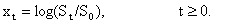(4)

The state variables  v1,t, v2,t , t>0, are the stochastic variances associated to xt, t>0,   Wt0,1, Wt0,2 ,Wt1, Wt2, t>0, are standard Wiener processes such that W00,1= W00,2=W01=W02=0,  dWt0,1, dWt0,2 ,dWt1, dWt2 are their stochastic differentials and  <dWt0,1dWt0,2 >= 0,  <dWt0,1dWt1 >= r0,1dt, <dWt0,2dWt2 >= r0,2dt,   <dWt1dWt2>=0 , where < × > denotes the mean of  × and r0,1, r0,2Î[-1,1] are  constants  known as correlation coefficients. The quantities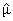,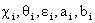, i=1,2 are real constants.  The fact that the model is a two scale stochastic volatility model is translated in the assumption that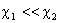. The equations (1), (2), (3)  must be equipped with an initial condition, that is:The random variables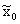,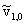,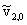are assumed to be concentrated in a point with probability one and we have=0. Later we will assume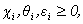,>0, i=1,2 and when we use the multiscale model we will choose ai=-1/2, bi=1, i=1,2.

We conclude this Section noting that when we evaluate  the option prices we use the no arbitrage pricing theory, that is we compute the option price as expected value of a discounted payoff with respect to an equivalent martingale measure, known as risk-neutral measure. The risk neutral measure is a probability measure equivalent to the statistical measure associated to the process (1), (2), (3) that guarantees that the discounted value of the underlying is a martingale. When we assume that the volatility is constant, the risk neutral measure is unique. In the case of one factor stochastic volatility models, such as the Heston model, this uniqueness does not hold anymore and we have a family of risk neutral measures parameterized by a real parameter called risk premium parameter. It is easy to see that in the case of the multiscale stochastic volatility model (1), (2), (3) we have a family of risk neutral measures parameterized by two risk premium parameters l1 and l2. This family of measures is given by the statistical measures associated to the following dynamical systems:equipped with the initial conditions (5), (6), (7), where ci*(li) = ci+li, q*i(li) = ciqi/(ci+li), i = 1,2, where ci, qi,  i=1,2 are the constants appearing in the stochastic differential system (1), (2), (3) and the real parameters li, i = 1,2 are the risk premium parameters. We impose c*i(li) ³ 0, q*i(li) ³ 0,        i =1,2, that is we impose li > -ci, i = 1,2.

In  we use the risk neutral  measure defined through (8), (9), (10)  to derive formulae involving  one dimensional integrals of explicitly known integrands  to price European call and put vanilla options. These formulae when we choose a1=0, a2=-1/2, b1=0, b2=1 price European vanilla options under the Heston model.  The formula derived in  for the price of a European vanilla call option under the Heston model improves the well known formula derived in  to price options under the Heston model (see formula (10) of  ). In fact the formula derived in   does not generalize naturally the Black Scholes formula since it is not the expected value of the discounted payoff computed using the risk neutral measure induced by the fundamental solution of the Fokker Planck equation associated to the Heston dynamical system. This is due to the fact that the measure used by Heston in   is not a probability density in the two variables log-return and stochastic variance at the initial time t=0 and, as a consequence, it is not a probability density function at time t, t > 0. The formula derived in   instead is truly analogous to the original Black Scholes formula in the context of the stochastic volatility models considered.

### 3. Calibration problem using synthetic data

The calibration problem that we solve consists in determining the values of the model parameters, of the correlation coefficients and of the initial stochastic variances that give the best approximation of the option prices used as data. We consider the approximation in the least squares sense so that we reformulate the calibration problem as a nonlinear constrained least squares problem.

In the numerical experiments proposed here and in Section 4 we choose  in equations (1), (2), (3)  a1 = a2 = -1/2 and b1 = b2 = 1. Let us denote with      Q = (e1,q1,r0,1,c1,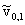,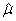,l1,e2,q2,r0,2,c2,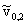,l2) the vector of the parameters of the multiscale model (including the risk premium parameters), of the correlation coefficients and of the initial stochastic variances and let m be a positive integer, we denote with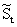Î R+ the price of the asset at time t, t ³ 0, with Ct(,Ti,Ki), i = 1,2,¼,m, the data, that is the prices at time t of the European vanilla call options having maturity time Ti and strike price Ki, i = 1,2,¼,m, and with  CMt,Q(,Ti,Ki), i = 1,2,¼,m, the prices of the same European vanilla call options obtained using formula (59) of  that prices European vanilla call options in the multiscale stochastic volatility model considered taking as maturity time t = Ti-t, i = 1,2,¼,m, and as asset price S0 =. When necessary we denote with CHt, Q(,Ti,Ki), i = 1,2,¼,m, and with CBt, Q(,Ti,Ki), i = 1,2,¼,m, the prices of the same options obtained using the Heston formula, that is the formula deduced from the multiscale formula when we choose a1 = 0 , b1 = 0, a2 = -1/2 and b2 = 1, and the Black Scholes formula respectively.

Note that CHt, Q and CBt, Q depend only on some of the components of the vector Q.  Please note that in the experiments presented in this website and in    we use as data only the prices of call options.  More general sets of data will be considered elsewhere.

Let R13 be the 13-dimensional real Euclidean space and let M be the set of the admissible vectors Q, that is:The constraints that define M translate some elementary properties of the model (1), (2), (3).

At time t, t ³ 0, the calibration problem considered is translated into the following nonlinear least squares problem:

 min Q Î M Lt( Q), t ³ 0,

(12)

where the objective function Lt( Q) is defined as follows: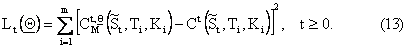When needed we solve problem (12) replacing CMt,Q with CHt,Q or with CBt,Q. The formulation of the calibration problem through (11), (12), (13) is only one formulation among many other possible formulations. It is known that the objective function (13) may have flat regions where the objective function changes slightly in correspondence of significant changes of the independent variables. So that special attention must be devoted to the choice of the initial guess of the iterative procedure used to solve the minimization problem (12). In fact in order to choose the initial guess of the minimization procedure we first explore the feasible region M taking a set of (feasible) random points and evaluating the objective function on this set of points. From these points we select a subset of points to be used as initial guesses. We solve the optimization problem (12) using a projected steepest descent method. This method is an iterative procedure that, starting from an initial feasible vector Q0, generates a sequence  {Qn}, n = 0,1,¼ of feasible vectors, Qn Î M, n = 0,1,¼, moving along a descent direction obtained via a suitable projection on the active constraints of minus the gradient with respect to Q of Lt. The optimization  procedure stops when the vector Qn generated satisfies for the first time the following criterion:

 Lt( Qn) £ etol,  or   n > nmax,

(14)

### where etol and nmax are positive constants that will be chosen later.

Let us present a numerical experiment using synthetic data (i.e.: synthetic option prices). This  experiment shows that the formulation of the calibration problem (11), (12), (13) is able to determine the model used to generate the synthetic option prices. We generate three sets of synthetic data, that is three sets of synthetic option prices. The first set is obtained generating the option prices with the Black Scholes formula choosing the volatility s equal to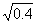and the risk free interest rate r equal to 0.03, the second one is obtained generating the option prices with the Heston model formula obtained using the formula derived in ,   and choosing the parameters a1 = 0, b1 = 0, a2 =-0.5, b2 = 1 and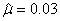, q2 = 0.5, c2 = 9.9, r2 = -0.06, e2 = 0.4, l2 =-0.1,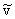2,0 = 0.5. The third one is obtained generating the option prices using the multiscale model formula contained  in   choosing, q1 = 0.8, q2 = 0.5, c1 = 1.5, c2 = 9.9, r1 = -0.04, r2 = -0.06, l1 = -0.05, l2 = -0.1, e1 = 0.4, e2 = 0.2,1,0 = 0.8,2,0 = 0.5. In the generation of the Heston and of the multiscale option prices we choose the risk free interest rate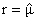. The synthetic data generated with the Black Scholes formula, with the Heston formula and with the multiscale formula  have been perturbed with an additive stochastic noise uniformly distributed of magnitude in absolute value at most equal to 1% of the absolute value of the datum perturbed.

We calibrate the multiscale model solving the constrained least squares optimization problem (12) with the variable metric steepest descent procedure announced previously choosing in (14) etol = 0.03 and nmax = 10000.

Figures 1, 2, 3 show the trajectories of the slow varying variance v1,t, t >0, and of the fast varying variance v2,t, t>0, obtained calibrating the model on the previous three sets of data and the “true” trajectories of the stochastic variances v1,t, v2,t, t >0, obtained integrating the Black Scholes model, the Heston model and the multiscale model with the “true” parameters,  correlation coefficients and  stochastic initial variances. Note that the “true” trajectories of the stochastic variances together with the corresponding “true” trajectories of the asset price are the trajectories  used to generate the synthetic data and that when we use the asset price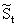at time  t>0  in (13) the quantities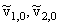coming  from the solution of the calibration problem (11), (12), (13) must be  interpreted as the stochastic variances at time t>0.

Figures 1, 2 show the results obtained using as data the option  prices generated by the Black Scholes and the Heston models respectively. These Figures show that the paths reconstructed with the calibration procedure of the stochastic variance v1,t, t > 0, are substantially constant and that the estimated values of v1,t, t > 0, are small. While the paths obtained solving the calibration problem using the third set of data, that is the data generated using the multiscale model, show that the calibration procedure works correctly (Figure 3). Furthermore in the case of the first set of data that is the data generated using the Black Scholes model (Figure 1) the reconstructed stochastic variance v2,t, t > 0, is substantially constant. This is in agreement with the well known properties of the Black Scholes model.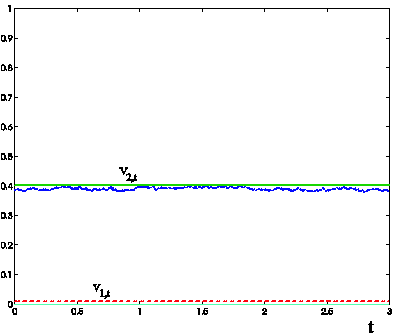Figure 1: Trajectories of the stochastic variances v1,t (red dotted line corresponding to a reconstructed c1 approximately equal to 9.96·10-4), v2,t (blue dotted line corresponding to a reconstructed c2 approximately equal to 7.71) obtained solving the calibration problem using as data the option prices generated with the Black Scholes model and trajectory of the ``true" Black Scholes variance (green line) versus time t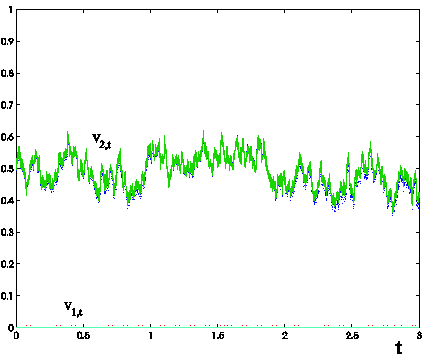Figure 2: Trajectories of the stochastic variances v1,t (red dotted line corresponding to a reconstructed c1 approximately equal to 7.93·10-3), v2,t (blue dotted line corresponding to a reconstructed c2 approximately equal to 7.68) obtained solving the calibration problem using as data the option prices generated with the Heston model and trajectory of the ``true" Heston variance (green line) versus time t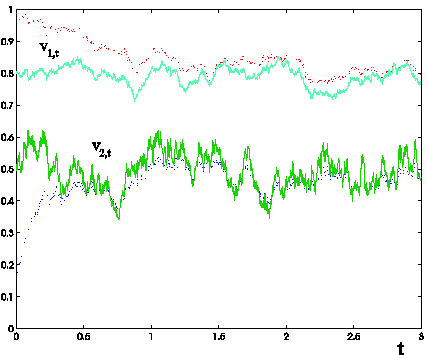Figure 3: Trajectories of the stochastic variances v1,t (red dotted line corresponding to a reconstructed c1 approximately equal to 8.84·10-1), v2,t (blue dotted line corresponding to a reconstructed c2 approximately equal to 5.5) obtained solving the calibration problem using as data the option prices generated with the multiscale model and trajectory of the ``true" stochastic variances of the multiscale model (green lines) versus time t

For the three models considered (Black Scholes, Heston, multiscale ) the following movies show a sample trajectory  of the log-return xt obtained using the vector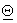obtained solving the calibration problem (12) and the same sample trajectory obtained using the  “true”  vectoras function of time. This “true” vector is the one  used to generate the data of the calibration problem.

 Black Scholes model Heston model Multiscale model

### 4. Calibration problem and forecasting experiments using real data

In this Section we propose some experiments  that use real data. We consider three models that is the Black Scholes model, the Heston model and the multiscale model (1),(2),(3). We study the values of the model parameters (including the risk premium parameters), of the correlation coefficients and of the initial stochastic variances implied by the observed prices (available to us) of the European vanilla call options on the S&P 500 index of the New York Stock Exchange in the year 2005 and we show that the multiscale stochastic volatility model captures satisfactorily the ``smile" effect. To avoid excessive numerical work we analyze the market data (i.e. S&P 500 index and the corresponding  option prices) only in the months of January, June and November, 2005. The results presented relative to these months are representative of the results obtainable from an exhaustive analysis of the 2005 data. We have solved the calibration problems relative to the Black Scholes model, to the Heston model and to the multiscale model, using the call option prices  of January 3, 2005, or of June 3, 2005 and or of November 3, 2005.  The formulation of the calibration problem for the Black and Scholes model and for the Heston model is analogous to the formulation (11), (12), (13) used for the multiscale model.  Note that for each model (Black Scholes, Heston, multiscale) we have solved the calibration problem three times (using as data the prices of January 3, June 3, November 3, 2005 respectively) and that since we use all the call option prices available the calibration procedure works simultaneously on out of money, at the money and in the money options. In the stopping criterion (14) of the optimization procedure we use etol=0.05 and nmax = 10000. We note that in some cases the optimization procedure stops because we have n > nmax, in these cases the smallest value of etol reached is approximately 0.07.

Once solved the calibration problems, we use the calibrated models to forecast the option prices in future days using the Black Scholes formula  or the Heston formula  or the multiscale model formula (see  for the explicit form of these formulae). In these formulae the asset price value used is the asset price value actually observed in the day of the forecast. We forecast the stochastic variances v1,t, v2,t, t > 0, using the mean values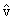1,t| Q,2,t| Q, t > > t0, conditioned to the estimates made at time t =t0 of the random variables v1,t, v2,t, t > t0, that we denote with F0 (i.e.:F0 = (1,0,2,0)) (see ,  for further details), that is using the formulae:where E( · ) is the expected value of  · , and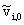, i = 1,2 are the initial stochastic variances at time t=t0 obtained solving the calibration problem. Note that in the analysis of the real data t=t0 corresponds to January 3, or to June 3, or to November 3, 2005, and that we can translate the origin of the time axis to t0.

Let nobs be a positive integer that is the number of call option prices at time t available in a given day, and let Ct(,Ti,Ki),  CBt, Q(,Ti,Ki),                 CHt, Q(,Ti,Ki), CMt, Q(,Ti,Ki) denote respectively the prices of European call options with strike price Ki and maturity time Ti (that corresponds to time to maturity ti = Ti-t) observed, generated by Black Scholes formula, generated by Heston formula  and generated  by multiscale formula, i=1,2,…, nobs. The following figures (i.e. Figures 4, 5, …,12) show the absolute error: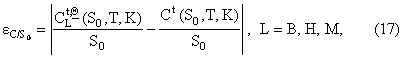obtained using the three models (L = B(Black Scholes), H(Heston), M(Multiscale)) as a function of the moneyness K/S0 = Ki/S0, i = 1,2,¼, nobs, when S0 =is the value of the S&P 500 index at the transaction day t considered and as a function of the time to maturity ti = Ti-t, i = 1,2,¼, nobs.

We use the parameters estimated on January 3, 2005 (nobs = 281) to forecast the option prices on January 7 (nobs=282), January 14 (nobs=283), January 28 (nobs=258), 2005 (see Figures  4, 5, 6). We use the parameters estimated on June 3, 2005 (nobs = 281) to forecast the option prices on June 7    (nobs= 288), June 14 (nobs = 291), June 28 (nobs = 278), 2005 (see Figures  7, 8, 9). We use the parameters estimated on November 3, 2005             (nobs = 303) to forecast the option prices on November 7 (nobs = 303), November 14 (nobs = 305), November 28 (nobs = 292), 2005 (see Figures 10, 11, 12)

Figures 4, 5,...,12 and the corresponding movies show the behaviour of the absolute error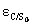committed forecasting the values of the option prices. We note that the bigger the time to maturity of the option considered is, the larger is the difference among the behaviour of the three models and the advantage of using the multiscale model when compared to the use of the Heston model or of the Black Scholes model.

The numerical experiment presented shows that the solutions of the calibration problem obtained using the data of January 3, 2005, June 3, 2005 and November 3, 2005 work well when used to forecast  the prices of the options in January 7, 14, 28, June 7, 14, 28 and November 7, 14, 28, 2005 respectively.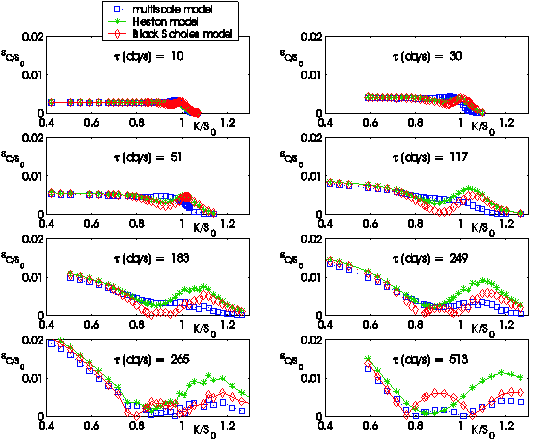Figure 4: January 7, 2005: Absolute error committed on the observed call option prices using the prices forecasted by the Black Scholes model, the Heston model and the multiscale model versus moneyness K/S0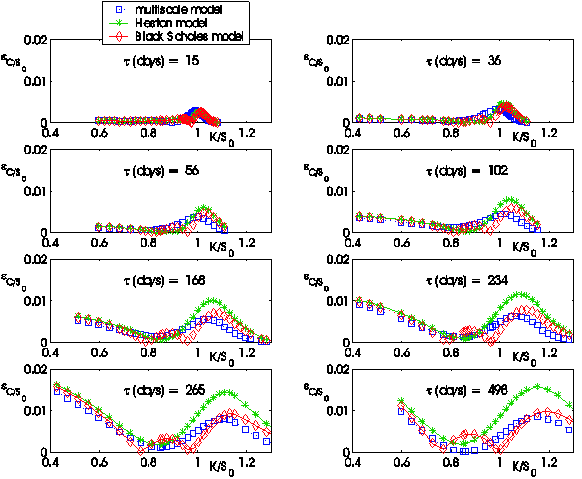Figure 5: January 14, 2005: Absolute error committed on the observed call option prices using the prices forecasted by the Black Scholes model, the Heston model and the multiscale model versus moneyness K/S0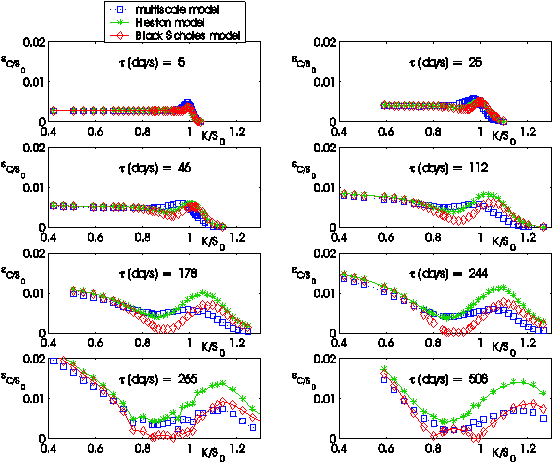Figure 6: January 28, 2005: Absolute error committed on the observed call option prices using the prices forecasted by the Black Scholes model, the Heston model and the multiscale model versus moneyness K/S0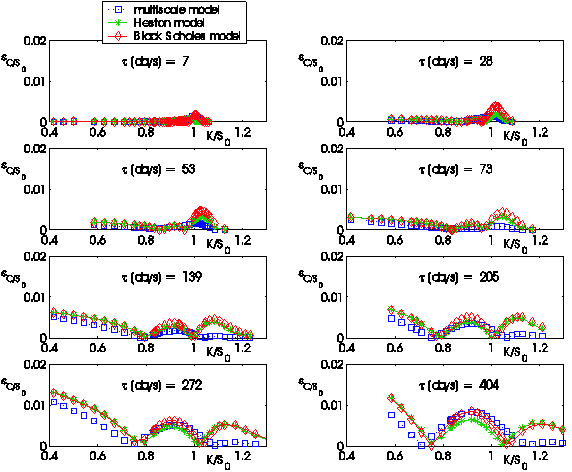Figure 7: June 7, 2005: Absolute error committed on the observed call option prices using the prices forecasted by the Black Scholes model, the Heston model and the multiscale model versus moneyness K/S0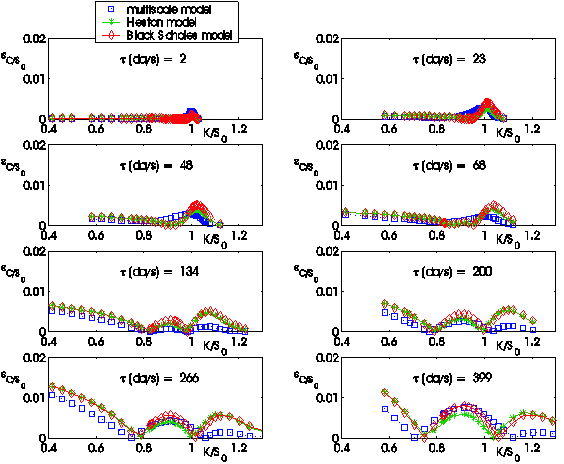Figure 8: June 14, 2005: Absolute error committed on the observed call option prices using the prices forecasted by the Black Scholes model, the Heston model and the multiscale model versus moneyness K/S0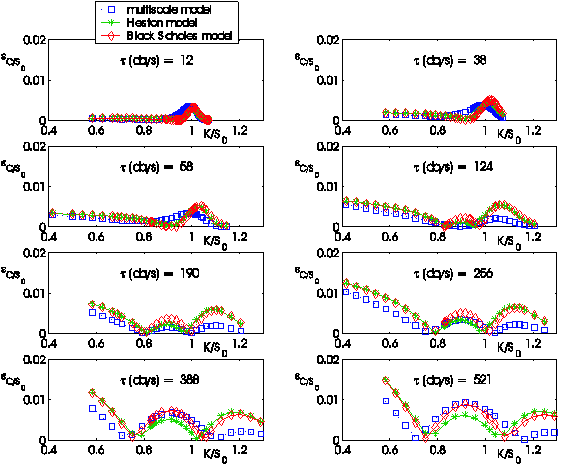Figure 9: June 28, 2005: Absolute error committed on the observed call option prices using the prices forecasted by the Black Scholes model, the Heston model and the multiscale model versus moneyness K/S0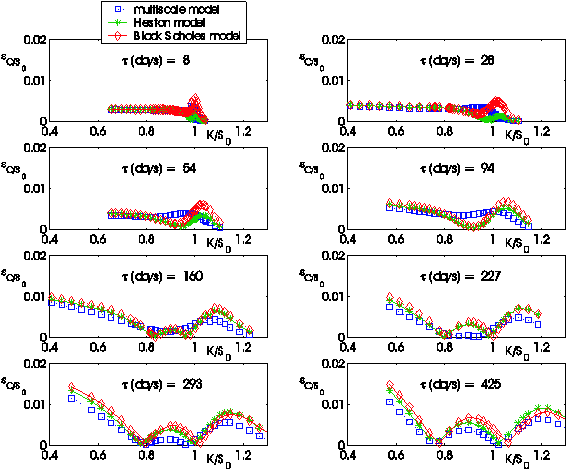Figure 10: November 7, 2005: Absolute error committed on the observed call option prices using the prices forecasted by the Black Scholes model, the Heston model and the multiscale model versus moneyness K/S0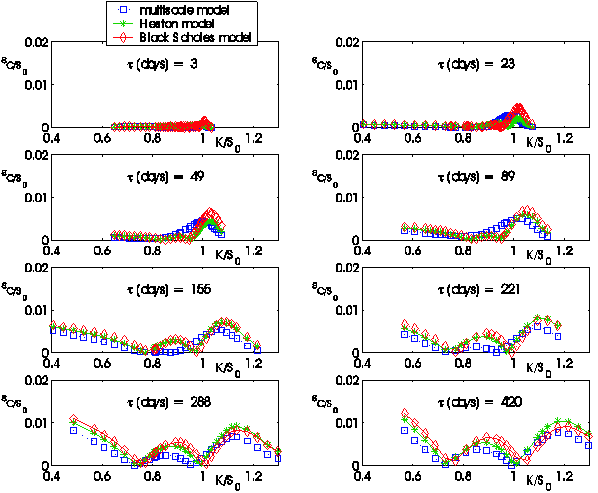Figure 11: November 14, 2005: Absolute error committed on the observed call option prices using the prices forecasted by the Black Scholes model, the Heston model and the multiscale model versus moneyness K/S0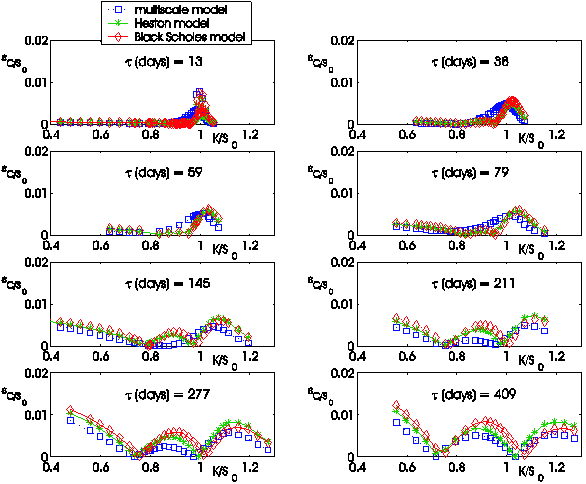Figure 12: November 28, 2005: Absolute error committed on the observed call option prices using the prices forecasted by the Black Scholes model, the Heston model and the multiscale model versus moneyness K/S0

5. References

     F. Black, M. Scholes, “The pricing of options and corporate liabilities”, Journal of Political Economy, 81, (1973), 637-659.

     R. Cont, P. Tankov,  Financial modelling with jump processes, Chapman & Hall/CRC Financial Mathematics Series, Boca Raton, Florida, USA, 2004.

  L. Fatone, F. Mariani, M.C. Recchioni, F. Zirilli: “An explicitly solvable multi-scale stochastic volatility model: option pricing and calibration”, Journal of Futures Markets, 29(9), (2009), 862-893.

    L. Fatone, F. Mariani, M.C. Recchioni, F. Zirilli: “The calibration of the Heston stochastic volatility model using filtering and maximum  likelihood  methods”, in Proceedings of Dynamic Systems and Applications, G.S.Ladde, N.G.Medhin, Chuang Peng, M.Sambandham Editors, Dynamic Publishers, Atlanta, USA, 5, (2008), 170-181, (http://www.econ.univpm.it/recchioni/finance/w6).

       L. Fatone, F. Mariani, M.C. Recchioni, F. Zirilli: “Maximum likelihood estimation of the parameters of a system of stochastic differential equations that models the returns of the index of some classes of hedge funds”, Journal of Inverse and Ill-Posed Problems, 15, (2007), 329-362, (http://www.econ.univpm.it/recchioni/finance/w5  contains downloadable software).

   J.P. Fouque, G. Papanicolaou, R. Sircar, K. Solna, Multiscale stochastic volatility asymptotics”, SIAM Journal on Multiscale Modeling and Simulation, 2, (2003), 22-42.

     S.L. Heston, “A closed-form solution for options with stochastic volatility with applications to bond and currency options”, Review of Financial Studies, 6, (1993), 327-343.

 A. Lipton, Mathematical methods for foreign exchange, World Scientific Pubblishing Co. Pte. Ltd, Singapore, 2001.

        F. Mariani, G. Pacelli, F. Zirilli: "Maximum likelihood estimation of the Heston stochastic volatility model using asset and option   prices: an application of nonlinear filtering theory",  Optimization Letters, 2, (2008), 177-222 , (http://www.econ.univpm.it/pacelli/mariani/finance/w1  contains downloadable software).

Entry n. 3576